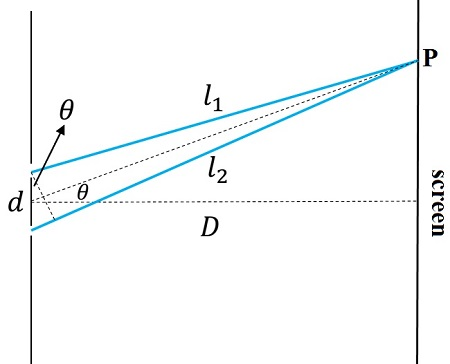# Light from a 550 nm source goes through two slits 0.040 mm apart, a screen is 1.5 m from the...

## Question:

Light from a 550 nm source goes through two slits 0.040 mm apart, a screen is 1.5 m from the slits.

a)what is the angular separation of the of the two first order maxima occurring on the screen?

b)what is the distance between the two first order maxima occurring on the screen?

c)what is the difference of the paths from the slits to a point on the screen where the fourth order maxima occurs.

## Young's experiment

The double-slit interference experiment due to Thomas Young was one of the first demonstrations of the wave nature of light. A beam of light goes through two narrow slits separated by a distance {eq}d {/eq}. By placing a screen behind an interference pattern can be obtained as a function of the path difference between the rays.

The setup is depicted below,Young studied under what conditions he obtained a maximum or a minimum at point {eq}\mathbf{P} {/eq}.

If the waves originated at each slit have optical paths {eq}l_1 {/eq} and {eq}l_2 {/eq}, the condition for a maximum is that both waves reach point {eq}\mathbf{P} {/eq} in phase:

if the path difference equals an integer number of wavelengths the waves are in phase and the result will be a maximum at point {eq}\mathbf{P} {/eq}.

On the other hand, if,

the path difference is a half-integer number of wavelengths the interference is destructive and results in a minimum at point {eq}\mathbf{P} {/eq}.

The path difference in Young's experiment is.

considering that the distance to the screen is much larger than the distance between the slits, {eq}D\gg d {/eq}. In this case, the angle {eq}\theta {/eq} is the same for both lines toward {eq}\mathbf{P} {/eq} and from the center of the setup.

a) Substituting equation (3) in expression (1) to determine the angular position of the maxima,

{eq}d\sin\theta=m\lambda {/eq}.

Since we are considering the first and second-order maxima, {eq}m=1, 2 {/eq},

{eq}\sin\theta_1 =\dfrac{\lambda}{d} {/eq}, for the first orden and,

{eq}\sin\theta_2 =\dfrac{2\lambda}{d} {/eq}, for the second-order.

Solving for {eq}\theta_1 {/eq} and {eq}\theta_2 {/eq},

hence,

{eq}\Delta \theta_{12}=\theta_2-\theta_1=0.79^\circ {/eq}.

The same result can be obtained without using the inverse trigonometric function. Given that the angles are very small the sine can be approximated as,

{eq}\sin\theta\sim \theta {/eq},

and the expression for the angular separation results in,

{eq}\Delta \theta=\dfrac{\lambda}{d}=0.01375 {/eq} rad, converting to degrees,

{eq}\Delta \theta=0.79^\circ {/eq}.

b) The distance between the slits can be obtained from the geometry of the experiment. The positions of the maxima are,

where we have used the tangent function of the angle {eq}\theta {/eq} defined as the position in the screen (associated to {eq}\theta {/eq}) measured from the center divided by the distance from the slits to the screen,

{eq}\tan\theta=\dfrac{y}{D} {/eq}.

Substituting, the distance between the maxima are,

{eq}\Delta y=y_2-y_1=D(\tan\theta_2-\tan\theta_1)\sim D(\sin\theta_2- \sin\theta_1)\sim D(\theta_2- D\theta_1) {/eq},

{eq}\Delta y=y_2-y_1=D\dfrac{\lambda}{d}=0.021 {/eq} m.

c) For the fourth-order maxima, we have from equation (1),

{eq}\Delta l_4=4\lambda {/eq}.

The path difference is,

{eq}\Delta l_4=4\cdot 550\times 10^{-9}\;m=2200 \times 10^{-9}\;m=2.2\times 10^{-6} {/eq} m.